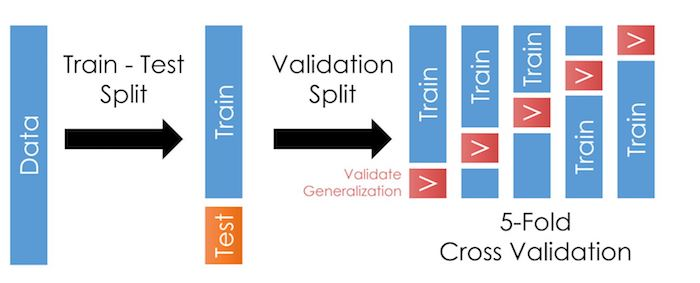# 5.4.1. Principles into Practice¶

Let’s put the principles from last chapter into code. Here is the pseudocode:

1. All of your import functions

3. Split your data into 2 subsamples: a “test” and “train” portion as in this page or this page or this page. This is the first arrow in the picture below.1 We will do all of our work on the “train” sample.

4. Before modelling, do EDA (on the training data only!)

• Sample basics: What is the unit of observation? What time spans are covered?

• Look for outliers, missing values, or data errors

• Note what variables are continuous or discrete numbers, which variables are categorical variables (and whether the categorical ordering is meaningful)

• You should read up on what all the variables mean from the documentation in the data folder.

• Visually explore the relationship between `v_Sale_Price` and other variables.

• For continuous variables - take note of whether the relationship seems linear or quadratic or polynomial

• For categorical variables - maybe try a box plot for the various levels?

• Now decide how you’d clean the data (imputing missing values, scaling variables, encoding categorical variables). These lessons will go into the preprocessing portion of your pipeline below. The sklearn guide on preprocessing is very informative, as this page and the video I link to therein.

5. Prepare to optimize a series of models (covered here)

1. Set up one pipeline to clean each type of variable

2. Combine those pipes into a “preprocessing” pipeline using `ColumnTransformer`

• The picture below illustrates 5 folds apparently based on the row number.

• There are many CV splitters available, including TimeSeriesSplit (a starting point for asset price predictions) and GroupTimesSeriesSplit is in development (which addresses a core problem with TimeSeriesSplit, and is shown in practice here)

3. Set up your scoring metric as discussed here

6. Optimize candidate model 1 on the training data

1. Set up a pipeline that combines preprocessing, estimator

2. Set up a hyper param grid

3. Find optimal hyper params (e.g. gridsearchcv)

4. Save pipeline with optimal params in place

7. Repeat step 6 for other candidate models

8. Compare all of the optimized models

```# something like...
for model in models:
cross_validate(model, X, y,...)
```

Here is that outline, but as a block of code you can use as a blueprint in projects:

```# import lots of functions
# split to test and train (link to split page/sk docs)

## pre-modeling (on the training data only!)

# do lots of EDA
# look for missing values, which variables are what type, and outliers
# figure out how you'd clean the data (imputation, scaling, encoding categorical vars)
# these lessons will go into the preprocessign portion of your pipeline

## optimize a series of models

# set up pipeline to clean each type of variable (1 pipe per var type)
# combine those pipes into "preprocess" pipe
# set up cv (can set up iterable to do OOS! or TimeSeriesSplit, or...)
# set up scoring

## optimize candidate model type #1:

#     set up pipeline (combines preprocessing, estimator)
#     set up hyper param grid
#     find optimal hyper params (gridsearchcv)
#     save pipeline with optimal params in place
#     (Note: you should spend time interrogating model predictions, plotting and printing.
#     Does the model struggle predicting certain obs? Excel at some?)

## optimize candidate model type #2

...

## optimize candidate model type #N

## compare the N optimized models

# build list of models (each with own optimized hyperparams)
# for model in models:
#    cross_validate(model, X, y,...)
# pick the winner!
```

1